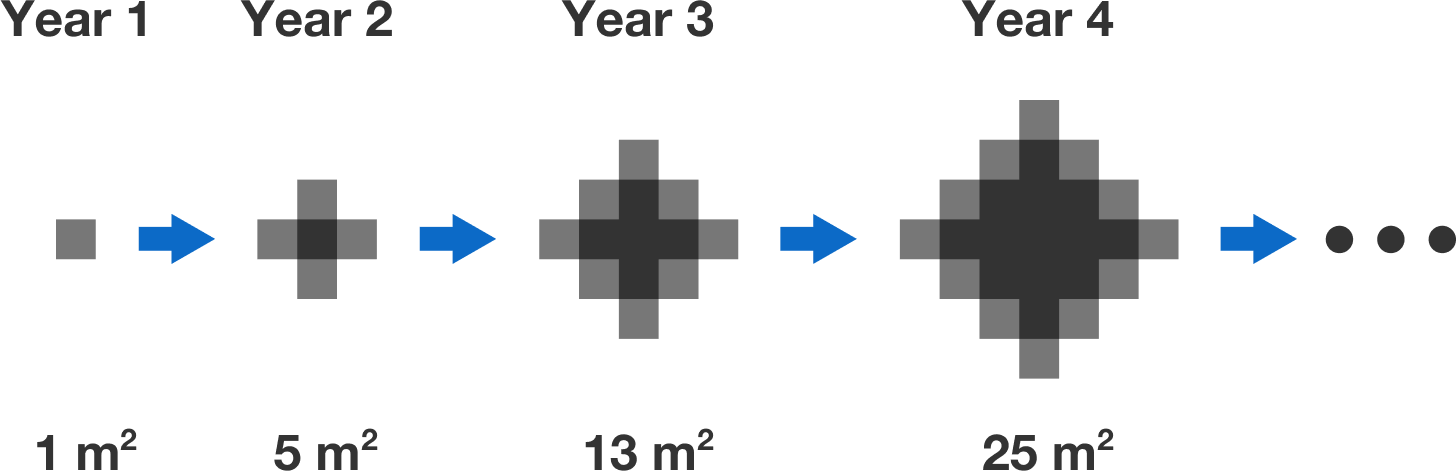# Don't fall in

Algebra Level 1

A sinkhole begins to open up in a deserted country. In the first year, the sinkhole is a $1\text{ m}$ square, and every year afterwards it grows an additional $1\text{ m}$ square from each of its outer edges.Steve notices the following pattern with the sinkhole area: \begin{aligned} 0^2+1^2 &= 1 \\ 1^2+2^2 &= 5 \\ 2^2+3^2 &= 13 \\ 3^2+4^2 &= 25. \end{aligned} Is the sinkhole area always the sum of the squares of two consecutive integers?

×